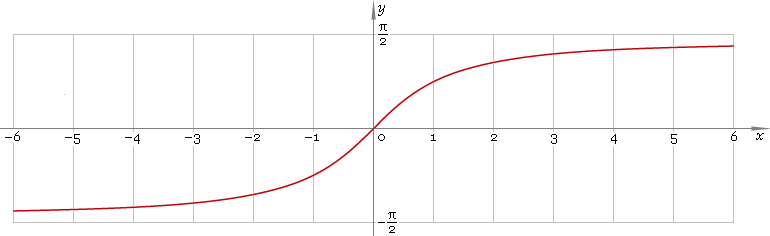The Art of Interface

# arctan or arctg — trigonometric arc tangent function

Category. Mathematics.

Abstract. Trigonometric arc tangent: definition, plot, properties, identities and table of values for some arguments.

## 1. Definition

Arc tangent is inverse of the tangent function.

## 2. Plot

Arc tangent is monotone antisymmetric function defined everywhere on real axis. Its plot is depicted below in fig. 1.Fig. 1. Plot of the arc tangent function y = arctanx.

Function codomain is limited to the range (−π/2, π/2).

## 3. Identities

Complementary angle:

arctanx + arccotx = π/2

and as consequence:

arctan cot φ = π/2 − φ

Negative argument:

arctan(−x) = −arctanx

Reciprocal argument:

arctan(1/x) = arccotx for x > 0,
arctan(1/x) = arccotx − π for x < 0

Sum and difference:

arctanx + arctany = arctan[(x + y) /(1 − xy)]
arctanx − arctany = arctan[(xy) /(1 + xy)]

Some argument values:

Argument xValue arctanx
00
2 − √3π/12
√(1 − 2 /√5)π/10
√2 − 1π/8
√3 /3π/6
√(5 − 2√5)π/5
1π/4
√(1 + 2 /√5)3π/10
√3π/3
√2 + 13π/8
√(5 + 2√5)2π/5
2 + √35π/12
Table 1. Arc tangent for some argument values.

## 4. Support

Trigonometric arc tangent function arctan or arctg of the real argument is supported by free version of the Librow calculator.

Trigonometric arc tangent function arctan or arctg of the complex argument is supported by professional version of the Librow calculator.

## 5. How to use

To calculate arc tangent of the number:

``arctan(-1);``

To calculate arc tangent of the current result:

``arctan(rslt);``

To calculate arc tangent of the number x in memory:

``arctan(mem[x]);``